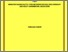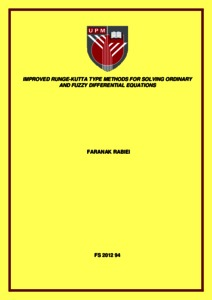# Improved Runge-Kutta type methods for solving ordinary and fuzzy differential equations

## Citation

Rabiei, Faranak (2012) Improved Runge-Kutta type methods for solving ordinary and fuzzy differential equations. Doctoral thesis, Universiti Putra Malaysia.

## Abstract

In this study, we constructed the Improved Runge-Kutta (IRK) type of methods for solving first and second order ordinary differential equations as well as fuzzy differential equations. With the aim to increase the computational efficiency of the methods, we obtained the methods of higher order with less number of stages or function evaluations. The methods which arise from the classical Runge-Kutta methods can also be considered as a special class of two-step methods, that is the approximation at the current point is based on the values or information from the two previous points. Hence, the methods contain the current internal stage k i as well as the previous internal stages k i. The aim here is to use the available internal stage in the previous step so that the resulting methods are more accurate. In the first part of the thesis, the order conditions of the methods are obtained using Taylor series expansion. Based on the order conditions, IRK methods of different orders and stages for solving first order ODEs are constructed. The convergence of the method is proven and the stability regions of the methods are also presented. Numerical results based on the new methods are compared with the existing methods in the literature showed that they are computationally more efficient. Next, the order conditions of the methods for solving some special second order ODEs are obtained using Taylor series expansion. Based on the order conditions as well as work done by Dormand (1996), Improved Runge Kutta Nystrom (IRKN) methods of different orders and stages for solving the special second order ODEs y00 = f(x; y) are constructed. The stability polynomial and stability region of of the methods are discussed. Numerical results based on the new methods are compared with the existing methods in the literature and it is showed that the new IRKN methods are computationally more efficient. We also derived IRKN methods which are specifically designed for the autonomous second order ODEs of the form y00 = f(y) based on the order conditions. These methods are called Accelerated Runge-Kutta Nystrom methods. The stability properties of the methods are discussed and numerical results showed that they are more efficient compared to the existing RKN methods. Finally, both IRK and IRKN methods are adapted for solving first and second order fuzzy differential Equations (FDEs). The convergence of IRK methods when applied to FDEs is also proven and numerical results proved that the IRK and IRKN methods give accurate results compared to the existing methods in the literature. In conclusion, the methods derived in this thesis are more efficient than existing methods for solving first and second order ordinary differential equations and fuzzy differential equations.Preview
Text
FS 2012 94 IR.pdfView Item